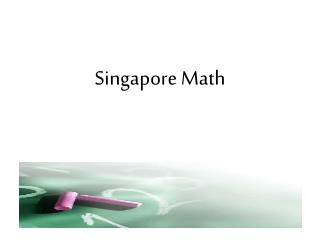Download PresentationSingapore Math

# Singapore Math

Download Presentation## Singapore Math

- - - - - - - - - - - - - - - - - - - - - - - - - - - E N D - - - - - - - - - - - - - - - - - - - - - - - - - - -
##### Presentation Transcript

1. Singapore Math

2. What is Singapore Math? • Emphasizes conceptualization before procedures (algorithms) • Builds understanding through part-whole thinking • Teaches computation methods that facilitate mental math • Reinforces understanding by providing practice through math stories and word problems.

3. What is Singapore Math? • Fewer topics are taught in greater depth. The goals are conceptualization and mastery. • Moving students through concrete (manipulatives) to pictorial (visual models) and then to abstract (symbolic) levels.

4. Three Foundational Areas That Support the Singapore Mathematics Curriculum Problem Solving Computation Taught by Place Value Mental Math

5. We teach different strategies to come up with the correct answer. Most of Singapore Strategies are Mental Math. Once students have established number sense, mental math, and problem solving, they learn to solve using algorithm. Students can use any strategy to solve any problem that they feel more comfortable with.

6. Addition Strategy Using Left to Right 24 + 33 = Add the tens Add the ones

7. Addition Strategy Using Left to Right 147 + 342 =

8. Addition Strategy Using Branch Method +

9. Number Bonds 6 7 1

10. d Adding Fractions 1 1 + 3 2

11. d Adding Fractions 1 1 + 3 2

12. d Adding Fractions 1 1 2 3

13. d Adding Fractions 1 1 + 3 2

14. d Adding Fractions 2 2 + 5 7

15. d Adding Fractions 2 2 + 5 7

16. d Adding Fractions 2 2 5 7

17. d Adding Fractions 2 2 + 5 7 24 = 35

18. AN EXAMPLE OF MODEL DRAWING Nora had 4 times as much money as Pedro. Melinda had 3 times as much money as Pedro. If Melinda had \$30, how much money did Nora have? American solution: Model-drawing solution: